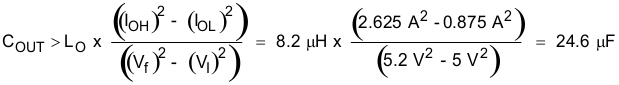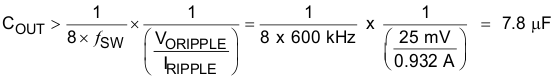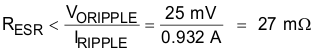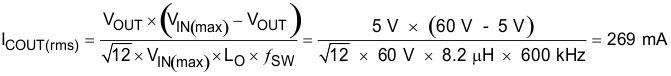JAJSBY0G August   2012  – June 2018

PRODUCTION DATA.

1. 特長
2. アプリケーション
3. 概要
1.     Device Images
4. 改訂履歴
5. Pin Configuration and Functions
6. Specifications
7. Detailed Description
1. 7.1 Overview
2. 7.2 Functional Block Diagram
3. 7.3 Feature Description
4. 7.4 Device Functional Modes
8. Application and Implementation
1. 8.1 Application Information
2. 8.2 Typical Application
1. 8.2.1 Design Requirements
2. 8.2.2 Detailed Design Procedure
3. 8.2.3 Application Curves
9. Power Supply Recommendations
10. 10Layout
1. 10.1 Layout Guidelines
2. 10.2 Layout Example
11. 11デバイスおよびドキュメントのサポート
12. 12メカニカル、パッケージ、および注文情報

• DDA|8
• DDA|8

#### 8.2.2.4 Output Capacitor

There are three primary considerations for selecting the value of the output capacitor. The output capacitor determines the modulator pole, the output voltage ripple, and how the regulator responds to a large change in load current. The output capacitance needs to be selected based on the most stringent of these three criteria.

The output capacitor must also be sized to absorb energy stored in the inductor when transitioning from a high to low load current. The catch diode of the regulator can not sink current so energy stored in the inductor can produce an output voltage overshoot when the load current rapidly decreases. A typical load step response is shown in Figure 35. The excess energy absorbed in the output capacitor will increase the voltage on the capacitor. The capacitor must be sized to maintain the desired output voltage during these transient periods. Equation 33 calculates the minimum capacitance required to keep the output voltage overshoot to a desired value, where LO is the value of the inductor, IOH is the output current under heavy load, IOL is the output under light load, Vf is the peak output voltage, and Vi is the initial voltage. For this example, the worst case load step will be from 2.625 A to 0.875 A. The output voltage increases during this load transition and the stated maximum in our specification is 4 % of the output voltage. This makes Vf = 1.04 × 5 = 5.2. Vi is the initial capacitor voltage which is the nominal output voltage of 5 V. Using these numbers in Equation 33 yields a minimum capacitance of
24.6 μF.

Equation 34 calculates the minimum output capacitance needed to meet the output voltage ripple specification, where ƒsw is the switching frequency, VORIPPLE is the maximum allowable output voltage ripple, and IRIPPLE is the inductor ripple current. Equation 34 yields 7.8 μF.

Equation 35 calculates the maximum ESR an output capacitor can have to meet the output voltage ripple specification. Equation 35 indicates the ESR should be less than 27 mΩ.

The most stringent criteria for the output capacitor is 29.2 μF required to maintain the output voltage within regulation tolerance during a load transient.

Capacitance de-ratings for aging, temperature and dc bias increases this minimum value. For this example, 2 x 47 μF, 10 V ceramic capacitors with 5 mΩ of ESR will be used. The derated capacitance is 58.3 µF, well above the minimum required capacitance of 29.2 µF.

Capacitors are generally rated for a maximum ripple current that can be filtered without degrading capacitor reliability. Some capacitor data sheets specify the Root Mean Square (RMS) value of the maximum ripple current. Equation 36 can be used to calculate the RMS ripple current that the output capacitor must support. For this example, Equation 36 yields 269 mA.

Equation 32.Equation 33.Equation 34.Equation 35.Equation 36.